WEBINAR
Efficient Green Energy: Exploring Simulation-Driven Design for High-Torque Wind Turbine Machine
Thursday, September 21, 2023
Time
SESSION 1
SESSION 2
CEST (GMT +2)
03:00 PM
08:00 PM
EDT (GMT -4)
09:00 AM
02:00 PM
HOME / Applications / Capacitance calculation for a parallel plate capacitor

# Capacitance calculation for a parallel plate capacitor

Used Tools:## What is a capacitor?

Capacitor  is an electrical device that can store electrical energy. In a way they are similar to batteries but not exactly the same. In a battery, it is the chemical reactions which produces electrons in one location (also called as a terminal in battery terminology) and the movement of these electrons from one terminal to another results in a current flow. But capacitors don’t produce electrons but only distributes them unevenly. A capacitor can be charged by connecting it to a battery. Once it is charged (and removed from the battery) it stores charges. You can now use this capacitor to say light up a small light bulb for some time. A simplest capacitor is a parallel plate capacitor (figure 1). It has 2 parallel metallic plates separated by a distance. If there is no material in between the plates, then it is filled with air. This air which is an insulator is also called as dielectric.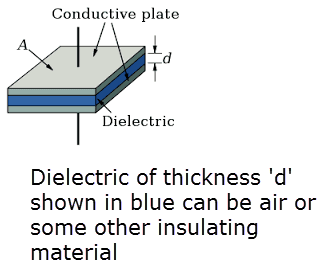Figure 1 - Parallel plate capacitor

## How does a capacitor store charges?

Let us refer to Figure 1. When the top plate is connected to the positive terminal of a battery and the bottom plate to the negative terminal, the capacitor is being charged. Before the capacitor is connected to the battery, it is electrically neutral. The amount of positive and negative charges in the top plate are the same. This is also the case for the bottom plate. So as a unit, the capacitor is electrically neutral (a state when the quantity of positive charge is the same as the negative charge). Now when connected to the battery, the electrons from the top plate move to the bottom plate through the wire and the battery. This creates a net positive charge on the top plate and a net negative charge on the bottom plate. Still as a unit, the capacitor (the 2 plates put together) is still electrically neutral. It is just that the charges are distributed in such a way that one plate contains a net positive charge and the other a net negative charge. Now this capacitor can be connected to a load like a light bulb and there will be a flow of electrons from the bottom plate to the top plate through the light bulb. The light bulb sees a current coming in and hence it glows. This flow of electrons happens till the top and bottom plate becomes electrically neutral. So you don’t expect the light bulb to be glowing for a long period of time.

## How are capacitors measured?

Capacitors are measured and rated by the amount of charge it can hold. This is measured in Capacitance and the unit for capacitance is Farads or Microfarads. Higher the capacitance, the more charge the capacitor can store.

## What role does the dielectric play?

So far we haven’t looked into dielectric and what role it plays in a capacitor.  The dielectric choice is important in a capacitor because as the capacitor is charged there is an electric field generated in the dielectric. When you put more and more charge, the electric field build up. This is possible up to a point when the electric field reaches the dielectric breakdown. At this point the dielectric breaks down and hence becomes a conductor of charges. The capacitor can no longer store charge.

## Our example – capacitor with multiple dielectric

Let us look at a case where there are multiple dielectrics in a capacitor. As shown in Figure 2, the parallel plates are filled with 3 dielectrics. The objective is to find the capacitance of this capacitor using EMS for SolidWorks.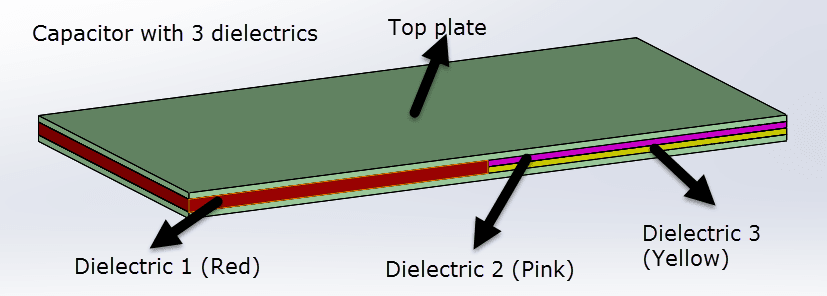Figure 2 - Capacitor with 3 dielectrics

EMS for Solidworks can

• Compute the capacitance of this capacitor

• Compute the electric field in the dielectric region

• Predict if any breakdown occurs

## Capacitance calculation EMS results

According to EMS, the value of the capacitance is 6e-11 Farads (Figure 3). Figure 4 shows the electric field distribution in the dielectric region when the capacitor is connected to 12 V battery.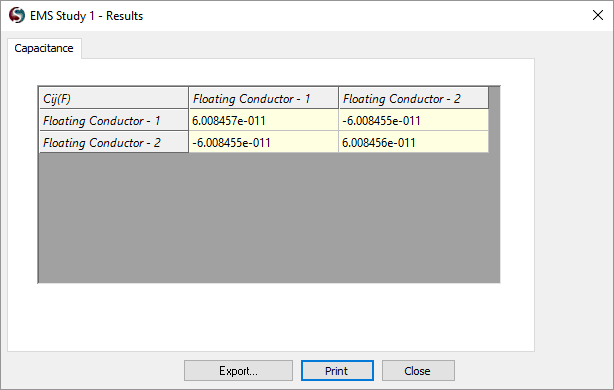Figure 3 - Capacitance as computed by EMS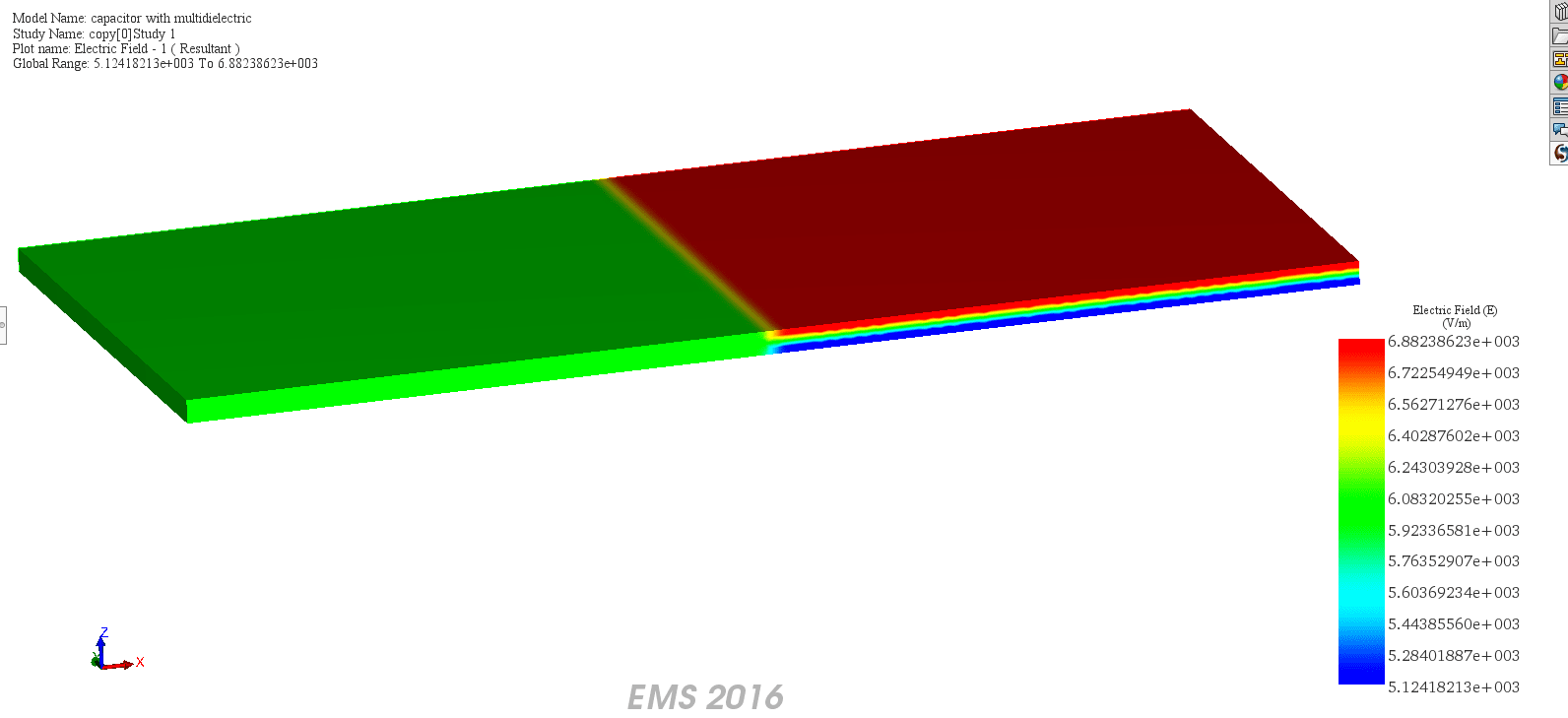Figure 4 - Electric field distribution in the dielectirc

## Validation

 Theoretical Result EMS Result Capacitance (F) 60.08e -12 F 60.084e -12 F

The formula for the theoretical result is taken from the source below.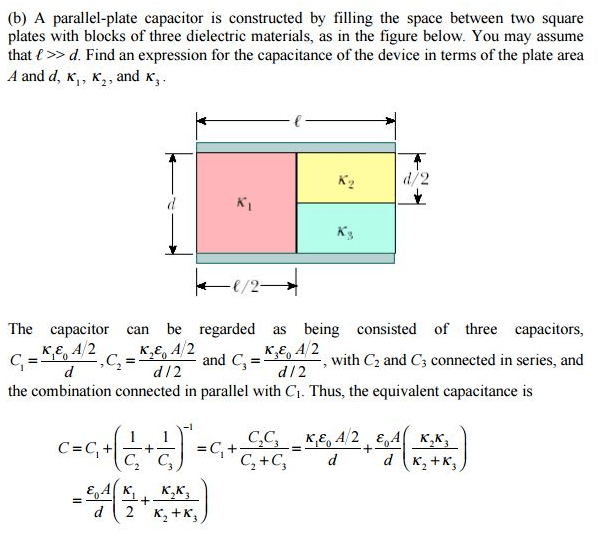## Conclusion

EMS for SolidWorks can accurately predict the capacitance and the electric field distribution of any type of capacitor. Most real life capacitors don’t have a plate area that is very very large. In fact, the length is very comparable to the thickness of the dielectric. In such cases, the analytical models don’t hold true. These are the cases where EMS for SolidWorks can be an excellent software for modeling and studying capacitors. The author wishes to express special thanks to Mr. Majdi El Fahim for the solid modeling and simulation of the capacitor presented in this article. For more information about EMWorks and its products, visit www.emworks.com.

## References

MIT OpenCourseWare - http://ocw.mit.edu (8.02SC Physics II: Electricity and Magnetism, Fall 2010)